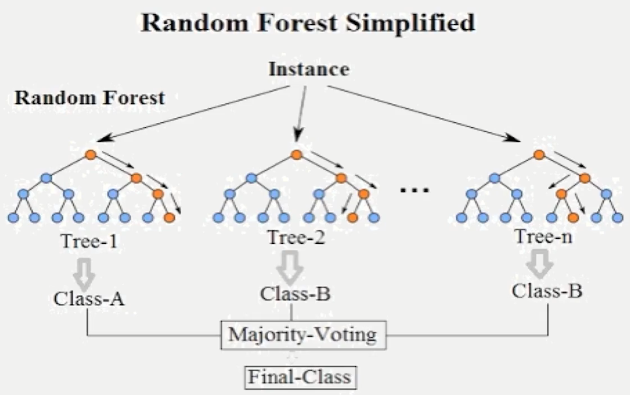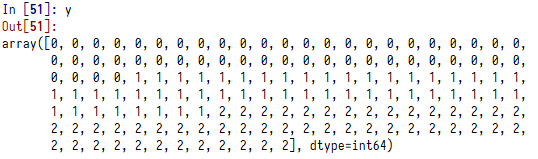# Python随机森林算法入门和性能评估from sklearn import ensemble
rf = ensemble.RandomForestClassifier(n_estimators=n)
rf = ensemble.RandomForestRegressor(n_estimators=n)

rf.fit(X,y)

## 1、导入相关的库

import pandas as pd
from sklearn.model_selection import cross_val_score
import numpy
from sklearn.preprocessing import LabelEncoder
from sklearn import linear_model
from sklearn import ensemble

## 2、准备数据

iris = pd.read_csv('http://archive.ics.uci.edu/ml/machine-learning-databases/iris/iris.data',header=None)
iris.columns=['SepalLengthCm','SepalWidthCm','PetalLengthCm','PetalWidthCm','Species']

le = LabelEncoder()
le.fit(iris['Species'])
features =  ['SepalLengthCm','SepalWidthCm','PetalLengthCm','PetalWidthCm']
X = iris[features]
y = le.transform(iris['Species'])## 3、建立模型

rf = ensemble.RandomForestClassifier(n_estimators=35)
rf.fit(X,y)
score = numpy.mean(cross_val_score(rf,X,y,cv=5,scoring='accuracy'))
print('平均性能得分：'+str(score))
print("特征重要性："+str(rf.feature_importances_))

## 4、和logistic分类进行性能对比

lm = linear_model.LogisticRegression()
score = numpy.mean(cross_val_score(lm,X,y,cv=5,scoring='accuracy'))
print('logistic回归模型平均性能得分：'+str(score))

logistic回归模型平均性能得分：0.96

## 5、回归

features =  ['SepalLengthCm','SepalWidthCm']
X = iris[features]
y = iris['PetalWidthCm']
rf = ensemble.RandomForestRegressor(n_estimators=15)
rf.fit(X,y)
score = numpy.mean(-cross_val_score(rf,X,y,cv=5,scoring='neg_mean_squared_error'))

print('随机森林回归模型平均性能得分：'+str(score))
print("随机森林回归模型特征重要性："+str(rf.feature_importances_))

##我们尝试用线性回归进行模型对比。
lm = linear_model.LinearRegression()
score = numpy.mean(-cross_val_score(lm,X,y,cv=5,scoring='neg_mean_squared_error'))
print('线性回归模型平均性能得分：'+str(score))

#### 请关注我的微信公众号